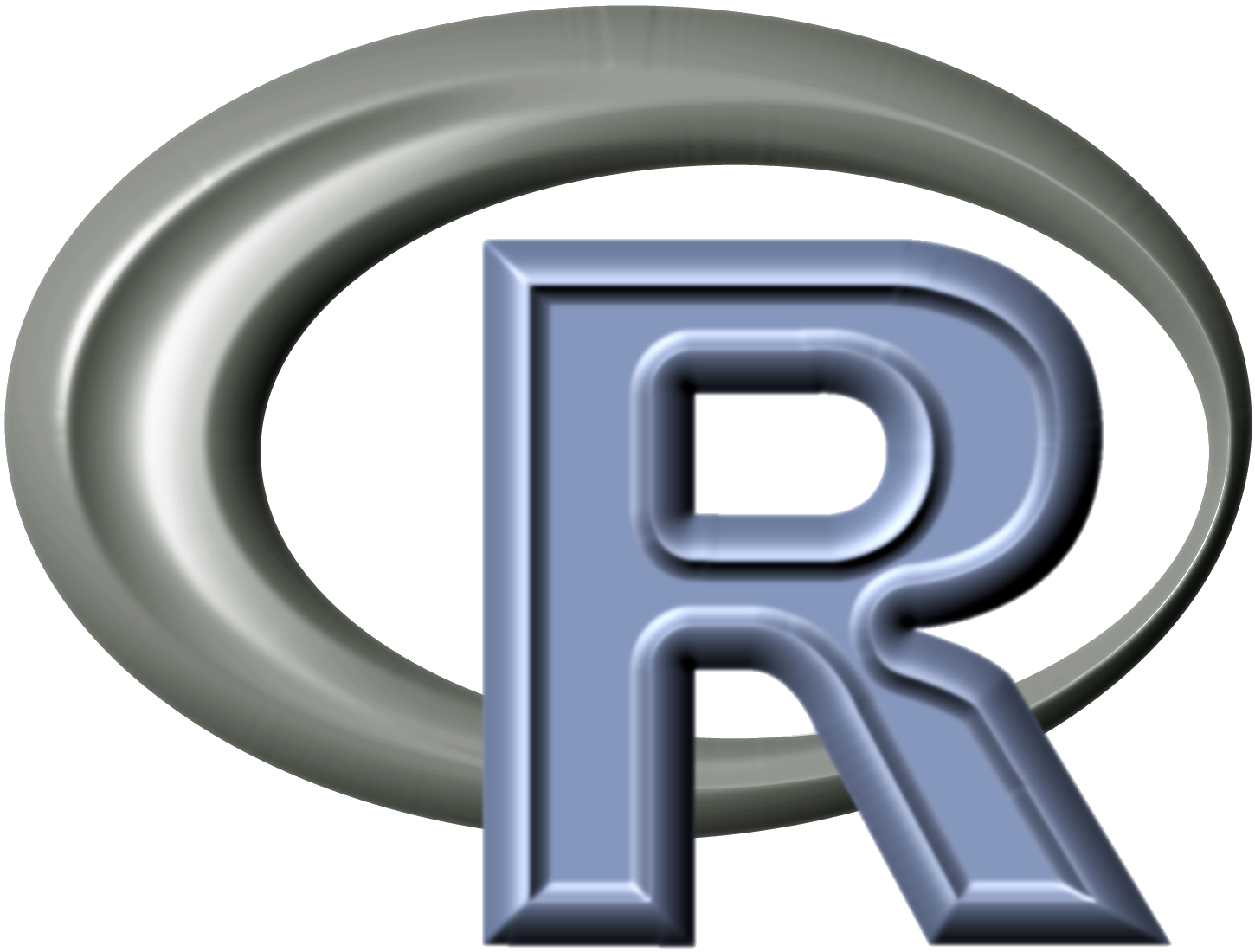# R for Numerical Analysis

Posted on Nov 21, 2012 in Computer Science

Things under legendu.net/outdated are outdated technologies that the author does not plan to update any more. Please look for better alternatives.1. Usually when we check whether two double values equal or not, we would check whether they're close enough or not. This is due to possible information loss of double values stored in computer. However, in R we can directly use `==` to check whether two objects (including double values) equal or not. This is because for double values, R will automatically check whether they are close or not instead of checking whether they are equal like other languages do.

2. Function `optim` and `optimize` can be used to find the minimum and maximum value of a function. By default these two functions find the minimum value. But we can use control=list(fnscale=-1) in optim and maximum=T in optimize to find the maximum value. Surely there are some difference between these functions. First we give an original value of the parameter for the function `optim` while we give an interval of the parameter for the function `optimize`. Second and also most important, the function `optimize` can only do the optimization over one variable while the function `optim` can do the optimization over multiple variables. However, when we use `optim` to do optimization over multiple variables, we must put these variables into a single vector.

3. We can use function `uniroot` to find the roots of a given function, and we can use function `optim` or `optimize` to find the maximum or minimum value of a function.